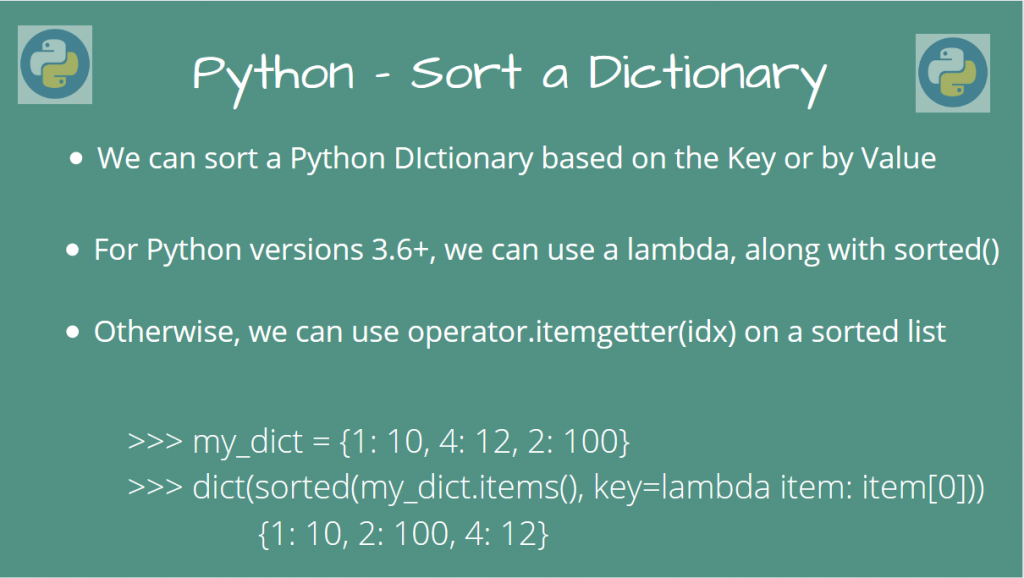# How to Sort a Dictionary in Python?There are different ways through which we can sort a Dictionary in Python. There are many ways, depending on whether you want to sort it by key or by value.

Let’s take a look at some of them in the article!

## Sort a Dictionary in Python by Value

If we want to sort a Dictionary in Python by value, there are a few ways using which we can achieve this.

### Method 1: Using sorted() using a lambda (Recommended for Python 3.6+)

On newer versions on Python (Python 3.6 and above), we can use a lambda on the `sorted()` method to sort a dictionary in Python.

We will sort the `dict.items()`, using the key as a lambda.

```my_dict = {1: 2, 2: 10, "Hello": 1234}

print({key: value for key, value in sorted(my_dict.items(), key=lambda item: item)})
```

Output

```{1: 2, 2: 10, 'Hello': 1234}
```

Indeed, we can see that our new dictionary has been sorted based on the value!

The reason why this method works on Python 3.6+ is that Dictionaries in the new versions of Python are now an ordered datatype.

This means that we can enumerate a dictionary as a list of items, and also perform operations that can change the order, such as sorting it.

But fear not. If you have an older version of Python, keep reading. We’ll show you another way to deal with this!

### Method 2: Using sorted() on older versions of Python

We can still use `sorted()` to sort the dictionary. But we something to make the Dictionary into an ordered type. The `operator` module has that, using `operator.itemgetter(idx)`.

The below snippet will sort our dictionary by value:

```import operator

my_dict = {1: 2, 2: 10, "Hello": 1234}

sorted_dict = sorted(my_dict.items(), key=operator.itemgetter(1))

print(sorted_dict)
```

More specifically, we form a sorted list using `sorted(dict.items()`), and pass `operator.itemgetter(1)` to it (Since the value is at index 1).

This will construct a callable that will grab that first element from the items list. We do this at every iteration, thereby getting a sorted dictionary!

## Sort a Dictionary in Python by Key

### Method 1: Use operator.itemgetter() (Recommended method for older versions of Python)

Now, if you want to sort a Dictionary by Key, we can use the `operator` method, like in the last section. The only change that we have to make is to sort the list based on keys now, so we call `operator.itemgetter(0)`.

```import operator

my_dict = {2: 10, 1: 2, -3: 1234}

# Sort the Dict based on keys
sorted_dict = dict(sorted(my_dict.items(), key=operator.itemgetter(0)))

print(sorted_dict)
```

Output

```{-3: 1234, 1: 2, 2: 10}
```

Indeed, the dictionary has been sorted based on the Key now!

### Method 2: Use sorted() with a lambda (Recommended method for Python 3.6+)

We can again use the `sorted()` method, with a lambda, on newer versions of Python.

Again, this is the same as before, but we’ll be now sorting based on value.

```my_dict = {2: 10, 1: 2, -3: 1234}
sorted_dict = dict(sorted(my_dict.items(), key=lambda item: item))
```

Output

```{-3: 1234, 1: 2, 2: 10}
```

Again, the output is the same as before.

## Conclusion

In this article, we learned how we could sort a Dictionary in Python; both by key and by value, using different approaches.